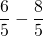Chapter 1: Algebra Review

# 1.2 Fractions (Review)

Working with fractions is a very important foundational skill in algebra. This section will briefly review reducing, multiplying, dividing, adding, and subtracting fractions. As this is a review, concepts will not be explained in as much detail as they are in other lessons. Final answers of questions working with fractions tend to always be reduced. Reducing fractions is simply done by dividing both the numerator and denominator by the same number.

Example 1.2.1

Reduce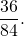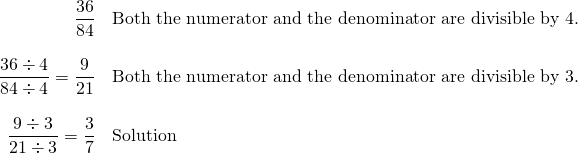The previous example could have been done in one step by dividing both the numerator and the denominator by 12. Another solution could have been to divide by 2 twice and then by 3 once (in any order). It is not important which method is used as long as the fraction is reduced as much as possible.

The easiest operation to complete with fractions is multiplication. Fractions can be multiplied straight across, meaning all numerators and all denominators are multiplied together.

Example 1.2.2

Multiply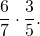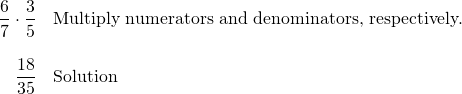Before multiplying, fractions can be reduced. It is possible to reduce vertically within a single fraction, or diagonally within several fractions, as long as one number from the numerator and one number from the denominator are used.

Example 1.2.3

Multiply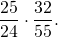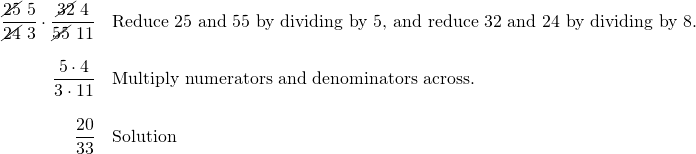Dividing fractions is very similar to multiplying, with one extra step. Dividing fractions necessitates first taking the reciprocal of the second fraction. Once this is done, multiply the fractions together. This multiplication problem solves just like the previous problem.

Example 1.2.4

Divide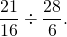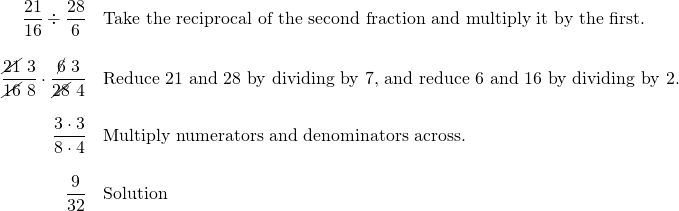To add and subtract fractions, it is necessary to first find the least common denominator (LCD). There are several ways to find the LCD. One way is to break the denominators into primes, write out the primes that make up the first denominator, and only add primes that are needed to make the other denominators.

Example 1.2.5

Find the LCD of 8 and 12.

Break 8 and 12 into primes: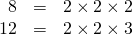The LCD will contain all the primes needed to make each number above.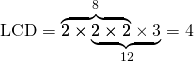Adding and subtracting fractions is identical in process. If both fractions already have a common denominator, simply add or subtract the numerators and keep the denominator.

Example 1.2.6

Add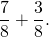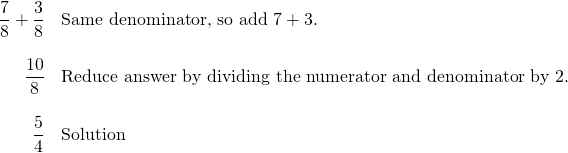While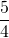can be written as the mixed number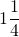, algebra almost never uses mixed numbers. For this reason, always use the improper fraction, not the mixed number.

Example 1.2.7

Subtract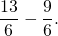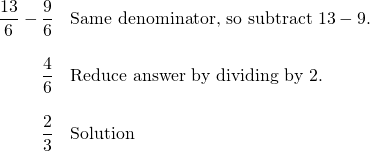If the denominators do not match, it is necessary to first identify the LCD and build up each fraction by multiplying the numerator and denominator by the same number so each denominator is built up to the LCD.

Example 1.2.8

Add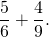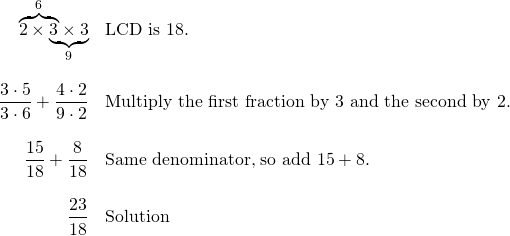Example 1.2.9

Subtract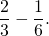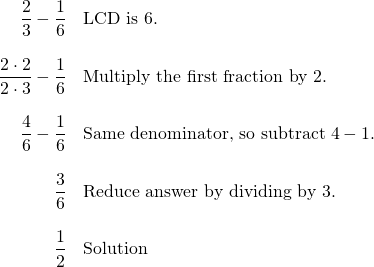# Questions

For questions 1 to 18, simplify each fraction. Leave your answer as an improper fraction.

1.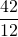2.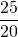3.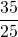4.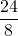5.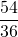6.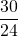7.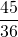8.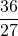9.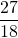10.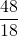11.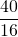12.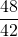13.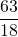14.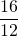15.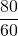16.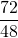17.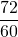18.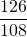For questions 19 to 36, find each product. Leave your answer as an improper fraction.

1.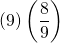2.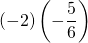3.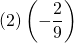4.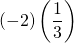5.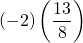6.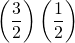7.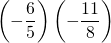8.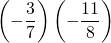9.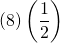10.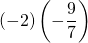11.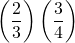12.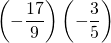13.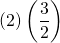14.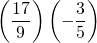15.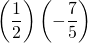16.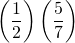17.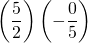18.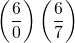For questions 37 to 52, find each quotient. Leave your answer as an improper fraction.

1.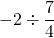2.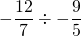3.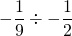4.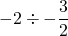5.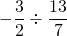6.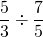7.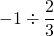8.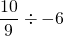9.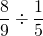10.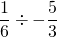11.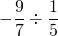12.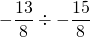13.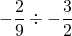14.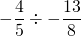15.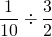16.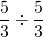For questions 53 to 70, evaluate each expression. Leave your answer as an improper fraction.

1.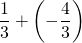2.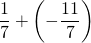3.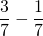4.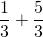5.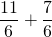6.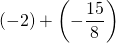7.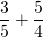8.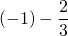9.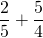10.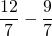11.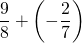12.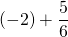13.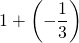14.15.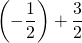16.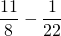17.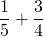18.